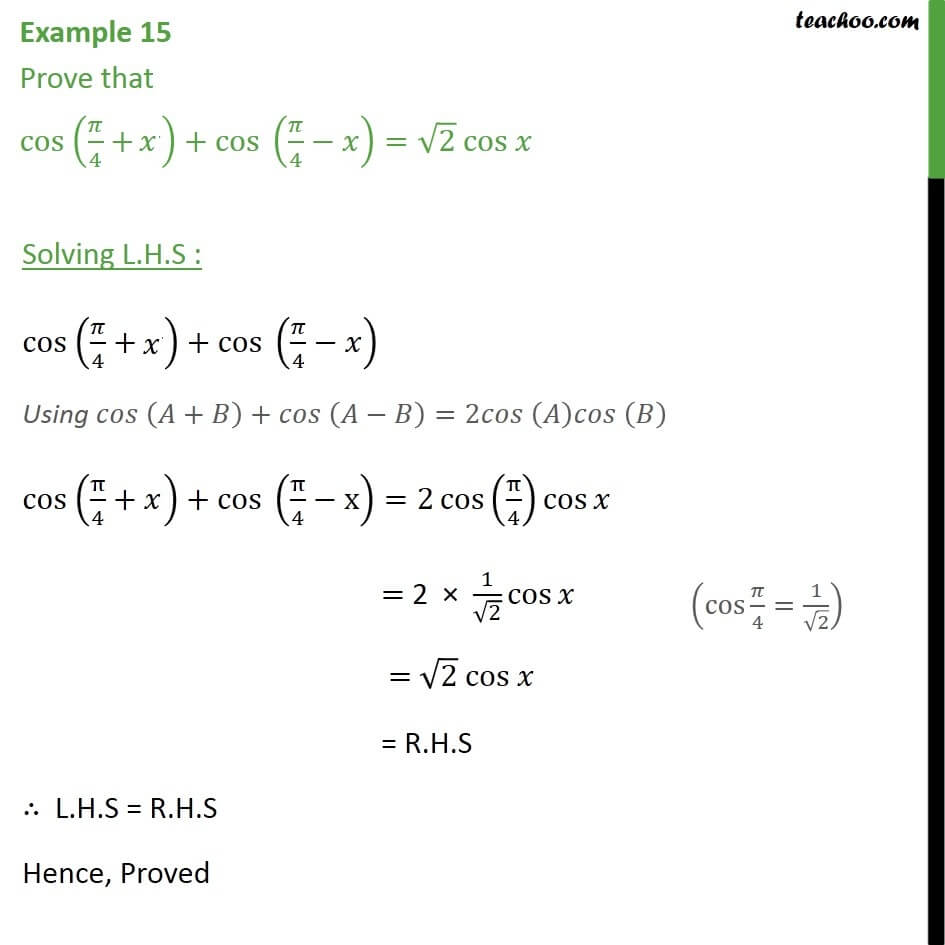1. Chapter 3 Class 11 Trigonometric Functions
2. Concept wise
3. 2x 3x formula - Finding value

Transcript

Example 15 Prove that cos (𝜋/4 + x) + cos (𝜋/4 − 𝑥) = root(2) cos 𝑥 Solving L.H.S : cos (𝜋/4 + x) + cos (𝜋/4 − 𝑥) Using 𝑐𝑜𝑠 𝐴+𝐵﷯+𝑐𝑜𝑠 𝐴−𝐵﷯=2𝑐𝑜𝑠 𝐴﷯𝑐𝑜𝑠 𝐵﷯ cos π﷮4﷯+π﷯+cos π﷮4﷯−x﷯=2 cos﷮ π﷮4﷯﷯﷯ cos﷮𝑥﷯ = 2 × 1﷮ ﷮2﷯﷯ cos﷮𝑥﷯ = ﷮2﷯ cos 𝑥 = R.H.S ∴ L.H.S = R.H.S Hence, Proved

2x 3x formula - Finding value